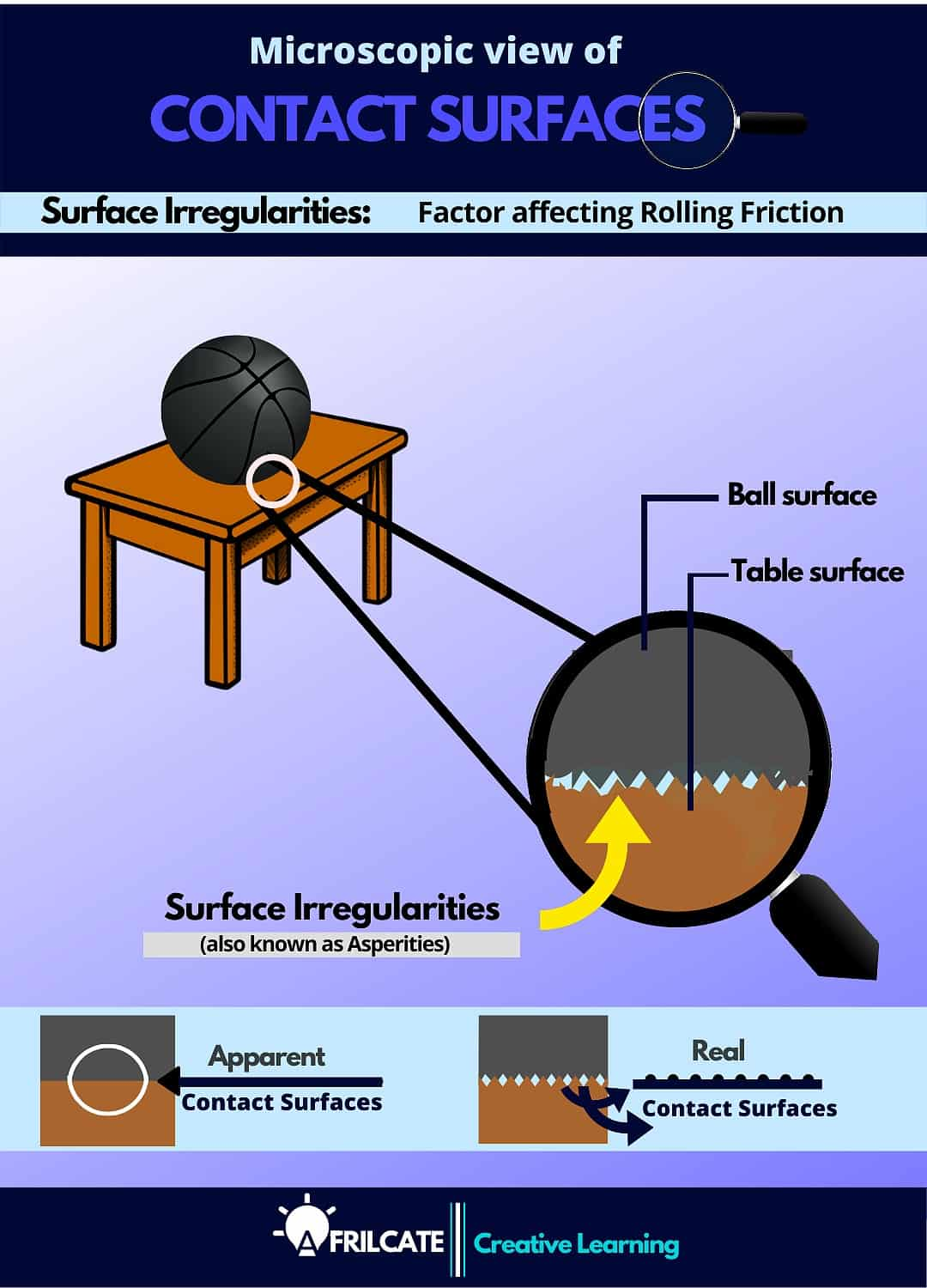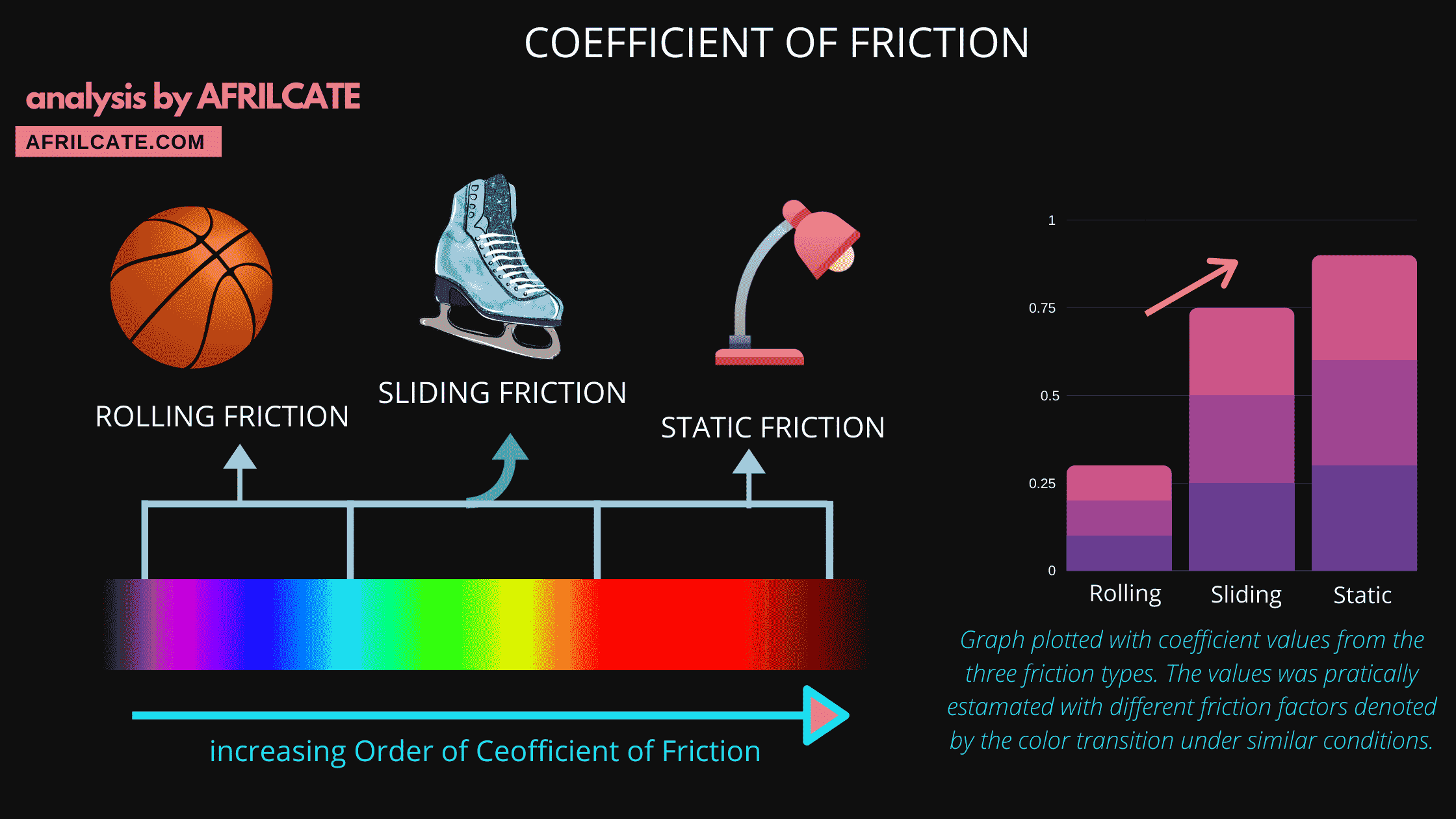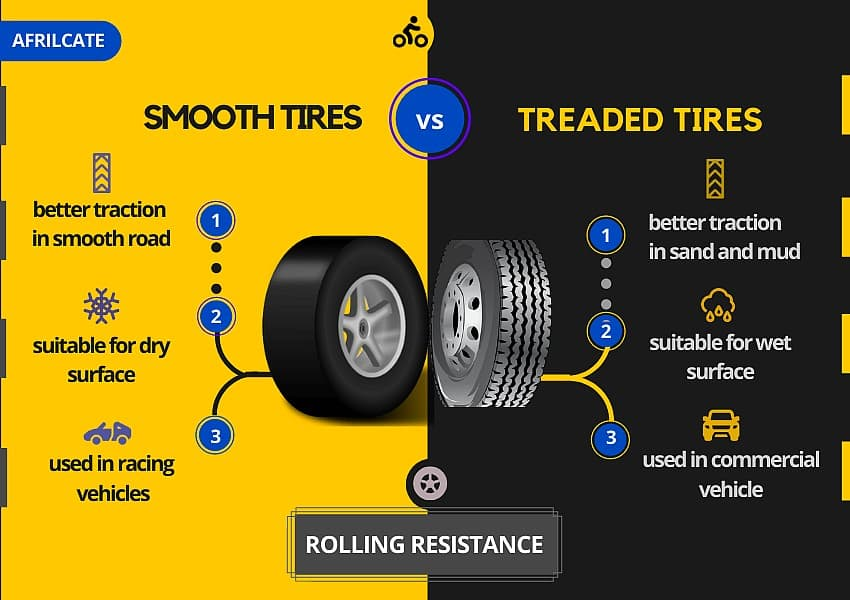Select Page

# Coefficient of Rolling Friction (Fully Explained)

written by Stanley Udegbunam ||updated Oct 23, 2020

# What is Coefficient of rolling friction?

The coefficient of rolling friction is a numerical value that quantifies the degree of motion resistance between a surface and a rolling object.

Also known as rolling friction coefficient, it measures the rolling resistance of an object against a surface.

A high coefficient of rolling friction indicates high rolling resistance between the given contact surfaces.

And a low coefficient of rolling friction indicates low rolling resistance between the given contact surfaces.

Recall that there are three types of dry friction: Static friction, kinetic friction, and rolling friction.

While static friction possesses the highest friction force, rolling friction accounts for the least friction force.

This is due to the minimal contact area of the rolling object and the surface beneath.As a result of this, the coefficient of rolling friction usually have the least value when compared with the coefficient of other friction types.Want to know more about rolling friction?, see our in-depth guideRolling Friction (The Complete Guide)

# Formula for Coefficient of rolling friction

Rolling friction coefficient is denoted with µk,r.

The formula for the coefficient of kinetic friction is given by;

 µk,r =  Fk,r /N

Where,

• Fk,r is the force of rolling friction
• N is the normal force, sometimes it’s denoted by η

The Normal force has the same magnitude as the weight.

It’s represented by the equation; N = m x g

If the object or plane is inclined at an angle (∅), the normal force becomes:

N = m gcos∅

Where m = mass of the object.

And g = acceleration due to gravity.

F and N are measured in units of force (such as Newton or pound).

Since friction is a force, the unit of the frictional force is the newton (N).

The coefficient of rolling friction is unitless.

# How to find the Coefficient of rolling friction

To find the coefficient of rolling friction, we will use the formula above to carry out some friction coefficient calculations.

## Calculations on Coefficient of rolling friction

### Example 1

A loaded trolley pushed on a wooden floor with μk,r = 0.2 has a mass of 40 kg.

What is the rolling resistance? Take g = 9.81m/s2

Solution

Fk,r​​k,r​ Fn

Normal force, Fn​ = mg

Coefficient of friction, μ k,r= 0.2

Fk,r​​k,r x mg

Fk,r​​= 0.2 x 40 x 9.81 = 78.48 N

### Example 2

Consider a milk tin rolling the floor.

Given N = 83 N and

Fk,r = 2.76 N,

What is the coefficient of the rolling friction?

Solution

Fk,r = µk,rN

µk,r = Fk,r/N

µk,r  = 2.76/83 = 0.033

Consider a car tire rolling on a smooth horizontal surface.

Given N = 806 N and

Fk,r = 1.38 N, the coefficient of the rolling friction is:

X wrong!!

✔ Correct

X wrong!!

X wrong!!

# Comprehensive List of Rolling friction Coefficients

The list below contains different rolling friction coefficient of various materials and surfaces in contact.

Because of the various factors affecting rolling friction, the coefficient of rolling friction is usually determined experimentally.

 COEFFICIENT OF ROLLING FRICTION OF DIFFERENT MATERIAL/CONTACT SURFACE railroad steel wheels on steel rails 0.001 - 0.002 rubber on concrete 0.35 - 0.45 nylon on concrete 0.03 - 0.04 bicycle tire on wooden track 0.001 bicycle tire on concrete 0.002 bicycle tire on asphalt road 0.004 bicycle tire on rough paved road 0.008 truck tire on asphalt 0.006 - 0.01 car tires on concrete, new asphalt, cobbles small new 0.01 - 0.015 car tires on tar or asphalt 0.02 car tires on gravel - rolled new 0.02 car tires on cobbles  - large worn 0.03 car tires on solid sand, gravel loose worn, soil medium hard 0.04 - 0.08 car tires on loose sand 0.2 - 0.4 Polyurethane 0.04 - 0.08
 CONTACT SURFACES COEFFICIENT OF ROLLING FRICTION railroad steel wheels on steel rails 0.001 - 0.002 rubber on concrete 0.35 - 0.45 nylon on concrete 0.03 - 0.04 bicycle tire on wooden track 0.001 bicycle tire on concrete 0.002 bicycle tire on asphalt road 0.004 bicycle tire on rough paved road 0.008 truck tire on asphalt 0.006 - 0.01 car tires on concrete, new asphalt, cobbles small new 0.01 - 0.015 car tires on tar or asphalt 0.02 car tires on gravel - rolled new 0.02 car tires on cobbles  - large worn 0.03 car tires on solid sand, gravel loose worn, soil medium hard 0.04 - 0.08 car tires on loose sand 0.2 - 0.4 Polyurethane 0.04 - 0.08

table extracted from engineering toolbox rolling resistance

# Frequently Asked Questions on Rolling Friction Coefficient

A short collected of common FAQ on the coefficient of rolling friction from the web.

The answers to the questions are all provided in the table above.

1. What’s the coefficient of rolling friction between a car tire and road surface?

Ans: The coefficient of rolling friction between car tires and road surface ranges from 0.01 – 0.02 depending on the nature of the road surface and the threaded nature of the tire.

Smooth/Slick tire offers better traction on dry surfaces and smooth roads.

While threaded tire offers better traction on wet surfaces and coarse/rough roads.rolling resistance of car tires

2. What’s the coefficient of rolling friction of steel and steel?

Ans: The coefficient of rolling friction of steel and steel ranges from 0.001 – 0.002.

3. What is the coefficient of rolling friction of rubber on concrete?

Ans: The coefficient of rolling friction of rubber on concrete ranges from 0.35 to 0.45.

4. What is the coefficient of rolling friction of nylon on concrete.

Ans: The coefficient of  rolling friction of nylon on concrete ranges from 0.03 to 0.04

5. What is the coefficient of rolling friction of Polyurethane?

Ans: The coefficient of rolling friction of Polyurethane ranges from 0.04 to 0.08 (refer to table)

# Now…Over to you

was it helpful in any way and would you like to see more content like this?

Share your thoughts and answers with us in the comment section below.